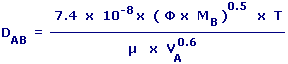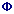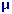Liquid Phase Diffusion Coefficient Calculator Enter value and click on calculate. Result will be displayed.DAB = Diffusion Coefficient of Liquid Phase         (Diffusion Coefficient of Solute A in Solvent B) MB = Molecular Weight Of Solvent B= Association Factor of Solvent B T = Absolute Temperature= Viscosity of Solvent B VA = Molal Volume of Solute A
 Enter your values: Association Factor of Solvent B: Molecular Weight Of Solvent B: Absolute Temperature: °K Viscosity of Solvent B: cP Molal Volume of Solute A: Cm3 /g-Mol Result: Diffusion Coefficient of Liquid Phase: Cm2 / S

 Web www.calculatoredge.com
 Diffusion can be defined as mixing of two or more substances or the net motion of a substance from an area of high concentration to an area of low concentration.

Diffusion Coefficient can be defined as a factor of proportionality representing the amount of substance diffusing across a unit area through a unit concentration gradient in unit time.

A number of correlations have been proposed for predicting the diffusion coefficient for a binary mixture of solute A in solvent B (e.g. propane in chlorobenzene). The Wilke-Chang method estimates this coefficient for dilute solutions.LINKSDISCLAIMERCONTACT US